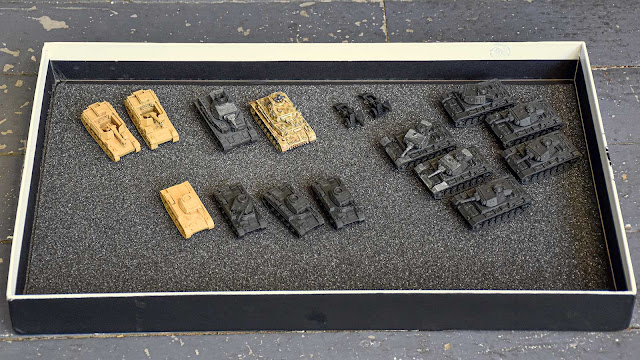### How To Draw A Hexagon

It's very, very easy. You will need a compass, a pen and/or pencil, and a ruler to make measurements and draw straight lines.

1. With the compass, draw a circle with the same radius as you want the length of the sides.
2. With the ruler, draw a line exactly bisecting the circle by going through the hole the compass made in the centre.
3. Draw two more circles with the compass, this time centring it at the points where the line bisects the circle.
4. With the ruler, connect the points where the three circles overlap.

And that's it. You have a perfect hexagon drawn to precise measurements.

If you need to draw a hexagon with measurements from side to side, you will need these ratios:

The circle will need to be 1.154 x the desired width of the hexagon, which means that the hexagon will be 0.86 x the diameter of the circle.

There you go. Easy-peasy.